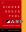Next Functional Programming in Perl 15

# Simple closure example

```        (* SML *)
fun mkcounter a' =
let val a  = ref a'
fun f = (a := !a + 1; print (toStr (!a)))
in f
end
```
```        # Perl
sub mkcounter {
my \$a = shift;
my \$f = sub { \$a++; print "\$a\n" };
return \$f;
}
```

 NextCopyright 2005 M. J. Dominus